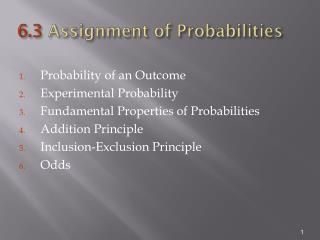# 6.3 Assignment of Probabilities - PowerPoint PPT PresentationDownload Presentation6.3 Assignment of Probabilities

6.3 Assignment of Probabilities
Download Presentation## 6.3 Assignment of Probabilities

- - - - - - - - - - - - - - - - - - - - - - - - - - - E N D - - - - - - - - - - - - - - - - - - - - - - - - - - -
##### Presentation Transcript

1. 6.3 Assignment of Probabilities • Probability of an Outcome • Experimental Probability • Fundamental Properties of Probabilities • Addition Principle • Inclusion-Exclusion Principle • Odds

2. Probability of an Outcome Let a sample space S consist of a finite number of outcomes s1, s2, … ,sN. To each outcome we associate a number, called the probability of the outcome, which represents the relative likelihood that the outcome will occur. A chart showing the outcomes and the assigned probability is called the probability distribution for the experiment.

3. Example Probability of an Outcome • Toss an unbiased coin and observe the side that faces upward. Determine the probability distribution for this experiment. • Since the coin is unbiased, each outcome is equally likely to occur.

4. Experimental Probability Let a sample space S consist of a finite number of outcomes s1, s2, … ,sN. The relative frequency, or experimental probability, of each outcome is calculated after many trials. The experimental probability could be different for a different set of trials and different from the probability of the events.

5. Example Experimental Probability Traffic engineers measure the volume of traffic on a major highway during the rush hour. Generate a probability distribution using the data generated over 300 consecutive weekdays.

6. Example Experimental Probability We will use the experimental probability for the distribution.

7. Fundamental Properties of Probabilities Let an experiment have outcomes s1, s2, … , sN with probabilities p1, p2, … , pN. Then the numbers p1, p2, … , pNmust satisfy: Fundamental Property 1 Each of the numbers p1, p2, … , pN is between 0 and 1; Fundamental Property 2 p1 + p2 + … + pN = 1.

8. Example Fundamental Properties Verify the fundamental properties for the following distribution. All probabilities are between 0 and 1 Total: 1.00

9. Addition Principle Addition Principle Suppose that an event E consists of the finite number of outcomes s, t, u, … ,z. That is E = {s, t, u, … ,z }. Then Pr(E) = Pr(s) + Pr(t) + Pr(u) + … + Pr(z), where Pr(E) is the probability of event E.

10. Example Addition Principle • Suppose that we toss a red die and a green die and observe the numbers on the sides that face upward. • Calculate the probabilities of the elementary events. • Calculate the probability that the two dice show the same number.

11. Example Addition Principle (2) • As shown previously, the sample space consists of 36 pairs of numbers • S = {(1,1), (1,2), … , (6,5), (6,6)}. • Each of these pairs is equally likely to occur. • The probability of each pair is • b)E = {(1,1), (2,2), (3,3), (4,4), (5,5), (6,6)}

12. Inclusion-Exclusion Principle Let E and F be any events. Then If E and F are mutually exclusive, then

13. Example Inclusion-Exclusion Principle A factory needs two raw materials. The probability of not having an adequate supply of material A is .05 and the probability of not having an adequate supply of material B is .03. A study determines that the probability of a shortage of both materials is .01. What proportion of the time will the factory not be able to operate from lack of materials?

14. Example Inclusion-Exclusion Principle (2) The factory will not operate 7% of the time.

15. Odds If the odds in favor of an event E are a to b, then On average, for every a + b trials, E will occur a times and E will not occur b times.

16. Example Odds In the two dice problem, what are the odds of rolling a pair with the same number on the faces? The probability of obtaining a pair with the same number on the faces is 1/6. The probability of not obtaining a pair with the same number on the faces is 5/6. The odds are

17. Summary Section 6.3 - Part 1 • A probability distribution for a finite sample space associates a probability with each outcome of the sample space. Each probability is a number between 0 and 1, and the sum of the probabilities is 1. The probability of an event is the sum of the probabilities of outcomes in the event.

18. Summary Section 6.3 - Part 2 • The inclusion-exclusion principle states that the probability of the union of two events is the sum of the probabilities of the events minus the probability of their intersection. If the two events are mutually exclusive, the probability of the union is just the sum of the probabilities of the events.

19. Summary Section 6.3 - Part 3 • We say that the odds in favor of an event are a to b if the probability of the event is a/(a + b). Intuitively, the event is expected to occur a times for every b times it does not occur.